### Halo 2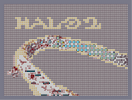Hover over the thumbnail for a full-size version.

Author capt_weasle author:capt_weasle n-art rated 2006-01-08 3 by 11 people. \$Halo 2#capt_weasle#none#00000000000000000000000000000000000000000000000000000000000000000000000000000000000000000000000000000000000000000000000000000000000000000000000000000000000000000000000000000000000000000000000000000000000000000000000000000000000000000000000000000000000000000000000000000000000000000000000000000000000000000000000000000000000000000000000000000000000000000000000000000000000000000000000000000000000000000000000000000000000000000000000000000000000000000000000000000000000000000000000000000000000000000000000000000000000000000000000000000000000000000000000000000000000000000000000000000000000000000000000000000000000000000000000000000000000000000000000000000000000000000000000000000000000000000000000000000000000000000|0^252,576!0^240,564!0^228,552!0^216,540!0^216,528!0^204,516!0^192,504!0^180,492!0^168,480!0^156,468!0^144,456!0^144,444!0^132,432!0^120,420!0^108,408!0^108,396!0^96,384!0^96,372!0^96,360!0^108,348!0^108,336!0^120,324!0^132,288!0^132,300!0^120,300!0^120,312!0^336,516!0^324,504!0^312,492!0^300,480!0^288,468!0^276,456!0^264,444!0^252,432!0^240,420!0^228,420!0^216,408!0^204,396!0^192,384!0^180,372!0^168,360!0^132,324!0^132,312!0^348,516!0^228,408!0^204,528!0^96,396!0^144,288!0^144,300!0^156,288!0^360,516!0^384,528!0^372,528!0^396,540!0^420,552!0^432,564!0^444,576!0^408,552!0^408,540!0^360,516!0^360,528!0^372,516!0^444,564!0^456,564!0^456,564!0^456,576!0^468,576!0^432,552!0^396,528!0^204,540!0^216,552!0^216,564!0^228,564!0^228,576!0^240,576!0^228,576!0^192,516!0^96,348!0^96,336!0^108,324!0^180,360!0^180,348!0^192,348!0^192,336!0^204,324!0^216,312!0^216,324!0^228,312!0^240,300!0^144,324!0^144,312!0^108,312!0^96,324!0^144,276!0^156,276!0^168,264!0^180,264!0^192,252!0^204,252!0^252,300!0^264,300!0^276,288!0^288,288!0^312,288!0^300,288!0^168,348!0^156,336!0^156,324!0^144,312!0^156,312!0^156,300!0^84,348!0^84,360!0^84,372!0^84,336!0^84,384!0^84,396!0^96,408!0^108,420!0^120,432!0^132,444!0^132,456!0^144,468!0^156,480!0^168,480!0^168,492!0^180,504!0^180,516!0^192,528!0^180,528!0^192,540!0^204,552!0^204,564!0^216,576!0^96,312!0^108,300!0^120,288!0^132,276!0^144,264!0^156,264!0^168,252!0^156,252!0^180,252!0^180,240!0^192,240!0^204,240!0^216,240!0^228,228!0^240,228!0^252,228!0^264,228!0^276,216!0^288,216!0^312,216!0^300,216!0^324,216!0^348,216!0^336,216!0^360,228!0^384,228!0^384,228!0^372,228!0^396,228!0^420,228!0^408,228!0^324,288!0^336,288!0^348,288!0^360,300!0^372,300!0^384,300!0^408,300!0^396,300!0^420,300!0^444,240!0^432,240!0^456,240!0^468,240!0^480,240!0^492,252!0^504,252!0^516,252!0^540,252!0^528,252!0^552,264!0^564,264!0^576,264!0^432,300!0^444,300!0^456,300!0^468,312!0^480,312!0^492,324!0^540,336!0^552,336!0^564,348!0^576,348!0^588,348!0^600,348!0^612,360!0^624,360!0^636,372!0^648,372!0^660,372!0^672,372!0^684,384!0^696,384!0^708,396!0^720,396!0^732,396!0^744,396!0^756,408!0^768,408!0^588,276!0^600,276!0^612,276!0^624,288!0^636,288!0^660,300!0^660,300!0^648,300!0^672,300!0^684,300!0^696,312!0^708,312!0^720,324!0^732,324!0^744,324!0^756,324!0^768,336!0^168,336!0^156,348!0^144,336!12^168,360!12^168,348!12^156,336!12^144,324!12^144,312!12^132,300!12^132,288!12^132,276!12^144,264!12^84,336!12^84,348!12^84,360!12^84,372!12^84,384!12^84,396!12^96,408!12^108,420!12^120,432!12^132,456!12^132,444!12^168,396!12^180,396!12^180,408!12^204,492!12^216,504!12^216,504!12^204,504!12^276,492!12^288,492!12^288,504!12^276,516!12^264,504!12^276,504!12^348,552!12^348,564!12^324,564!12^324,576!12^336,564!12^336,576!12^360,564!12^360,552!12^372,552!12^372,540!12^384,540!12^384,552!12^240,432!12^228,432!12^240,444!12^228,444!12^216,456!12^228,456!12^156,372!12^120,360!12^132,384!12^144,372!12^132,372!4^216,450,1!4^204,426,1!4^156,426,1!4^168,426,1!4^192,426,1!4^168,426,1!4^180,426,1!4^264,498,1!4^324,570,1!4^348,570,1!4^324,546,1!4^336,546,1!4^348,546,1!4^360,546,1!4^408,570,1!4^384,570,1!4^396,570,1!4^252,570,1!4^264,570,1!4^276,570,1!4^276,498,1!4^282,498,1!4^294,498,1!4^228,450,1!4^234,450,1!4^240,450,1!4^210,498,1!4^192,498,1!4^198,498,1!12^210,498!12^198,498!12^192,498!12^204,504!12^216,504!12^228,504!12^270,504!12^276,504!12^288,504!12^288,498!12^264,492!12^258,492!12^318,546!12^312,546!12^324,534!12^330,540!12^330,552!12^318,552!12^348,552!12^342,552!12^342,546!12^354,540!12^372,546!12^384,540!12^372,570!12^348,558!12^318,558!12^312,570!12^288,570!12^312,558!12^300,552!12^300,540!12^312,534!12^174,444!12^192,444!12^198,456!12^192,462!12^186,462!12^186,450!12^192,456!12^192,450!12^174,462!12^180,474!12^198,474!12^192,468!12^174,450!12^216,468!12^204,450!12^204,462!12^210,438!12^198,438!12^186,438!12^216,480!12^198,468!12^210,474!12^186,468!12^198,456!12^204,456!12^186,450!12^198,456!12^198,462!12^192,462!12^186,456!12^198,450!12^264,468!12^252,492!12^258,468!12^240,492!12^264,528!12^270,540!12^252,522!12^336,516!12^306,522!12^324,540!12^414,564!12^414,564!12^324,546!12^288,540!12^276,534!12^270,528!12^276,522!12^258,522!12^246,468!12^258,462!12^234,438!12^228,432!12^228,432!12^234,432!12^234,432!12^174,408!12^168,396!12^168,396!12^150,372!12^138,372!12^138,378!12^126,372!12^126,366!12^114,390!12^126,402!12^132,420!12^132,414!12^156,408!12^156,426!12^156,420!12^156,420!12^78,408!12^90,408!12^102,420!12^96,420!12^90,414!12^96,408!12^90,402!12^84,402!12^78,420!12^72,420!12^60,426!12^60,444!12^48,444!12^48,432!12^78,432!12^96,420!12^90,438!12^84,450!12^78,450!12^84,462!12^108,444!12^102,438!12^90,420!12^102,456!12^72,432!12^90,426!12^78,426!12^90,426!12^90,414!12^90,414!12^90,402!12^102,408!12^114,420!12^102,414!12^90,402!12^96,414!12^84,408!12^90,402!12^222,288!12^228,288!12^234,288!12^234,276!12^228,276!12^240,282!12^234,282!12^246,276!12^246,270!12^252,270!12^258,270!12^264,282!12^252,276!12^264,276!12^276,270!12^276,270!12^276,270!12^270,270!12^276,282!12^288,276!12^288,270!12^282,270!12^282,276!12^156,288!12^150,294!12^144,294!12^144,288!12^144,276!12^150,270!12^138,288!12^144,300!12^150,312!12^156,300!12^156,282!12^162,270!12^174,258!12^186,252!12^198,246!12^360,276!12^372,270!12^372,258!12^366,258!12^366,270!12^372,276!12^384,270!12^384,258!12^384,252!12^396,246!12^396,240!12^408,240!12^402,258!12^396,276!12^324,228!12^336,228!12^348,228!12^342,234!12^342,234!12^330,240!12^336,240!12^342,222!12^336,216!12^330,216!12^318,216!12^342,216!12^354,216!12^360,222!12^366,222!12^372,228!12^378,228!12^390,228!12^372,228!12^366,228!12^360,228!12^342,222!12^324,216!12^300,216!12^294,216!0^330,216!10^180,300!10^198,300!10^192,300!10^192,288!10^186,288!10^186,300!10^198,288!10^210,294!10^204,300!10^204,294!10^210,282!10^222,294!10^210,300!10^204,312!10^198,312!10^186,312!10^180,306!10^174,312!10^192,318!10^186,324!10^198,324!10^186,336!10^180,342!10^174,330!10^174,318!10^168,324!10^162,318!10^168,306!10^168,294!10^174,288!10^168,288!10^174,270!10^186,282!10^174,276!10^162,276!10^192,270!10^192,264!10^186,276!10^204,264!10^216,264!10^216,276!10^228,264!10^228,240!10^240,240!10^240,252!10^228,252!10^252,288!10^240,264!10^276,252!10^264,240!10^264,252!10^252,252!10^300,240!10^312,216!10^324,252!10^300,276!10^324,276!10^348,264!10^360,240!10^288,264!10^288,228!10^768,396!10^768,384!10^756,372!10^768,360!10^768,372!10^756,348!10^768,336!10^756,396!10^744,384!12^198,294!12^198,312!12^186,306!12^204,300!12^192,294!12^186,312!12^168,300!12^174,282!12^210,258!12^228,258!12^240,252!12^264,252!12^282,252!12^294,228!12^324,246!12^180,372!12^180,360!12^162,354!12^168,336!12^162,324!12^156,324!12^150,342!12^144,336!12^414,546!12^426,546!12^432,558!12^420,552!12^414,552!12^438,564!12^450,570!12^450,570!12^438,570!12^462,570!12^468,570!12^456,564!12^474,576!12^414,546!12^402,546!12^402,540!6^420,252,2,0,0,0!6^444,252,2,0,0,0!6^468,252,2,0,0,0!6^420,276,2,0,0,0!6^444,276,2,0,0,0!6^468,276,2,0,0,0!6^468,300,2,0,0,0!6^492,300,2,0,0,0!6^492,276,2,0,0,0!6^516,276,2,0,0,0!6^516,300,2,0,0,0!0^516,324!0^504,324!0^528,330!6^540,300,2,0,0,0!6^564,276,2,0,0,0!6^540,276,2,0,0,0!6^540,324,2,0,0,0!6^564,300,2,0,0,0!6^564,324,2,0,0,0!6^588,324,2,0,0,0!6^588,300,2,0,0,0!6^612,300,2,0,0,0!6^612,324,2,0,0,0!6^612,348,2,0,0,0!0^612,348!0^612,324!0^612,300!0^588,324!0^564,324!0^588,300!0^564,300!0^564,276!0^540,300!6^636,300,2,0,0,0!6^636,324,2,0,0,0!6^636,348,2,0,0,0!6^660,348,2,0,0,0!6^660,324,2,0,0,0!6^684,324,2,0,0,0!6^684,348,2,0,0,0!6^372,252,2,0,0,0!6^372,276,2,0,0,0!6^396,276,2,0,0,0!6^348,252,2,0,0,0!6^348,276,2,0,0,0!6^348,228,2,0,0,0!6^300,252,2,0,0,0!6^324,276,2,0,0,0!6^252,252,2,0,0,0!0^324,276!0^300,252!0^348,228!0^348,246!0^348,252!0^348,276!0^378,258!0^372,252!0^378,276!0^372,276!12^234,270!12^258,252!12^264,240!12^246,246!12^246,240!12^276,264!12^216,264!12^216,276!12^204,282!12^300,252!12^288,240!12^312,234!12^318,258!12^300,270!12^336,264!12^372,294!12^396,294!12^408,288!12^504,264!0^432,288!0^420,288!0^444,288!0^456,288!0^480,264!0^480,252!0^492,264!0^516,264!0^540,264!0^528,264!0^492,312!0^504,312!0^528,312!0^516,312!0^528,324!0^564,336!0^576,336!0^588,336!0^600,336!0^552,288!0^504,288!0^480,288!0^576,288!0^588,288!0^600,288!0^624,288!0^636,348!0^636,324!0^636,300!0^660,324!0^660,348!0^684,348!0^684,324!12^636,360!12^648,360!12^624,336!12^624,312!12^648,312!12^660,312!12^480,288!12^504,288!12^492,264!12^552,288!12^528,312!12^516,312!12^528,324!12^588,336!12^576,348!12^564,348!10^762,384!10^762,372!10^768,360!10^768,348!10^762,354!10^750,372!10^732,372!10^744,360!10^744,342!10^732,342!10^726,360!10^714,384!10^702,372!10^714,360!10^708,342!10^702,324!10^720,342!10^732,360!10^750,378!10^732,390!10^762,372!10^762,360!10^756,348!10^756,336!10^750,336!10^726,342!10^726,360!10^690,372!10^678,360!10^666,360!10^702,360!10^690,348!10^696,336!10^720,372!10^732,330!10^684,312!0^156,348!0^156,336!0^144,336!0^144,324!0^156,324!0^144,312!0^144,300!0^132,300!0^132,300!0^144,288!0^144,276!0^96,168!0^108,168!0^108,156!0^120,156!0^120,168!0^120,144!0^108,144!0^108,132!0^120,132!0^120,120!0^108,120!0^108,108!0^120,108!0^120,108!0^96,108!0^132,132!0^144,132!0^156,132!0^156,108!0^132,144!0^144,144!0^156,144!0^156,120!0^168,120!0^168,132!0^168,144!0^168,156!0^156,156!0^168,168!0^156,168!0^180,168!0^192,156!0^204,144!0^216,144!0^216,120!0^228,120!0^228,108!0^240,120!0^252,132!0^264,144!0^276,156!0^276,168!0^288,168!0^264,168!0^264,156!0^252,144!0^240,144!0^228,144!0^240,132!0^192,168!0^204,156!0^300,168!0^300,156!0^300,144!0^300,132!0^300,120!0^288,108!0^300,108!0^312,120!0^312,132!0^312,144!0^312,156!0^312,168!0^324,168!0^336,168!0^348,168!0^360,168!0^360,156!0^348,156!0^360,144!0^372,132!0^384,132!0^396,120!0^408,108!0^420,108!0^432,108!0^444,108!0^456,108!0^468,120!0^480,132!0^480,144!0^468,156!0^456,168!0^444,168!0^432,168!0^420,168!0^408,168!0^396,156!0^408,156!0^456,156!0^468,144!0^468,132!0^456,120!0^408,120!0^396,132!0^432,144!0^432,132!0^444,132!0^420,144!0^420,132!0^504,120!0^528,108!0^540,108!0^552,108!0^564,120!0^564,132!0^552,156!0^528,168!0^540,168!0^552,168!0^516,120!0^564,168!0^576,168!0^516,132!0^588,168!0^564,144!0^528,120!0^564,108!0^576,120!0^576,132!0^576,144!0^564,156!0^600,168!0^528,132!0^588,156!0^600,156!0^600,144!0^516,168!0^540,276!10^492,576!10^504,576!10^528,576!10^480,576!10^516,576!10^540,576!10^552,576!10^564,576!10^576,576!10^588,576!10^600,576!10^612,564!10^612,576!10^624,576!10^636,576!10^648,576!10^660,576!10^672,576!10^672,576!10^696,576!10^684,576!10^720,576!10^720,576!10^732,576!10^756,576!10^768,576!10^744,576!10^708,576!10^768,564!10^768,552!10^768,540!10^768,528!10^768,516!10^756,504!10^768,492!10^768,504!10^768,480!10^768,468!10^768,456!10^768,444!10^768,432!10^768,420!10^768,324!10^768,312!10^768,300!10^768,288!10^768,264!10^768,276!10^768,252!10^768,228!10^768,228!10^768,216!10^768,240!10^768,204!10^768,192!10^768,180!10^768,168!10^768,156!10^768,144!10^768,132!10^768,120!10^768,108!10^768,96!10^768,84!10^768,72!10^768,72!10^768,48!10^768,60!10^768,36!10^768,24!10^756,24!10^732,24!10^744,24!10^708,24!10^720,24!10^708,24!10^684,24!10^696,24!10^672,24!10^648,36!10^648,24!10^660,24!10^648,24!10^636,24!10^624,24!10^612,24!10^600,24!10^588,24!10^564,24!10^576,24!10^552,24!10^540,24!10^528,24!10^528,24!10^516,24!10^516,24!10^504,24!10^480,24!10^492,24!10^468,24!10^456,24!10^444,24!10^420,24!10^432,24!10^408,24!10^384,24!10^396,24!10^372,24!10^360,24!10^336,24!10^348,24!10^324,24!10^312,24!10^300,24!10^288,24!10^276,24!10^264,24!10^252,24!10^240,24!10^228,24!10^216,24!10^24,24!10^36,24!10^48,24!10^48,24!10^72,24!10^60,24!10^72,24!10^84,24!10^108,24!10^96,24!10^120,24!10^132,24!10^144,24!10^156,24!10^180,24!10^192,24!10^204,24!10^168,24!10^24,36!10^24,48!10^24,60!10^24,72!10^24,72!10^24,84!10^24,96!10^24,108!10^24,120!10^24,144!10^24,132!10^24,156!10^24,180!10^24,168!10^24,192!10^24,204!10^24,216!10^24,228!10^24,240!10^24,252!10^24,264!10^24,276!10^24,288!10^24,300!10^24,324!10^24,312!10^24,336!10^24,360!10^24,348!10^24,384!10^24,372!10^24,384!10^24,396!10^24,420!10^24,432!10^24,408!10^36,432!10^24,432!10^24,444!10^24,456!10^24,456!10^24,468!10^24,480!10^36,492!10^24,504!10^24,492!10^24,516!10^24,528!10^24,540!10^24,552!10^24,564!10^24,576!10^36,576!10^48,576!10^60,576!10^72,576!10^84,576!10^96,576!10^108,576!10^120,576!10^144,576!10^156,576!10^132,576!10^168,576!10^192,576!10^204,576!10^180,576!10^192,564!10^192,552!10^180,552!10^180,564!10^156,564!10^168,552!10^168,564!10^144,564!10^156,552!10^132,552!10^156,552!10^132,564!10^144,552!10^132,552!10^108,552!10^120,564!10^132,552!10^120,552!10^96,552!10^108,564!10^96,564!10^96,564!10^84,564!10^72,564!10^72,564!10^60,564!10^48,564!10^36,564!10^36,552!10^48,552!10^60,552!10^72,552!10^84,552!10^36,540!10^48,540!10^60,540!10^72,540!10^84,540!10^96,540!10^120,540!10^108,540!10^132,540!10^144,540!10^156,540!10^168,540!10^180,540!10^168,528!10^156,528!10^144,528!10^120,528!10^132,528!10^108,528!10^108,528!10^96,528!10^72,528!10^84,528!10^60,528!10^48,528!10^36,528!10^36,516!10^48,516!10^72,516!10^60,516!10^84,516!10^108,516!10^96,516!10^120,516!10^132,516!10^156,516!10^144,504!10^168,516!10^168,504!10^156,504!10^144,516!10^156,492!10^144,492!10^132,492!10^132,504!10^108,504!10^36,504!10^48,504!10^60,504!10^84,504!10^96,504!10^108,504!10^120,504!10^72,504!10^60,492!10^48,492!10^84,492!10^72,492!10^96,492!10^108,492!10^120,492!10^144,480!10^132,480!10^120,480!10^108,480!10^84,480!10^96,480!10^72,480!10^60,480!10^48,480!10^36,480!10^36,468!10^48,468!10^60,468!10^72,468!10^84,468!10^96,468!10^108,468!10^108,468!10^120,468!10^132,468!10^120,456!10^120,444!10^108,432!10^108,456!10^60,456!10^72,456!10^48,456!10^36,456!10^36,444!10^96,456!10^72,444!10^84,444!10^96,444!10^36,408!10^36,420!10^48,408!10^48,408!10^48,408!10^48,420!10^60,420!10^60,408!10^72,408!10^72,396!10^60,396!10^48,396!10^36,396!10^36,384!10^48,384!10^60,384!10^72,384!10^72,372!10^60,372!10^48,372!10^36,372!10^36,360!10^48,360!10^60,360!10^72,360!10^72,348!10^48,348!10^36,348!10^60,348!10^60,336!10^72,336!10^48,324!10^36,336!10^36,312!10^48,336!10^36,324!10^48,312!10^60,324!10^60,312!10^72,324!10^72,312!10^84,312!10^84,324!10^84,300!10^72,300!10^60,300!10^48,300!10^36,300!10^36,300!10^36,288!10^48,288!10^60,288!10^72,288!10^96,288!10^96,300!10^84,288!10^108,288!10^108,276!10^96,276!10^84,276!10^72,276!10^60,276!10^48,276!10^36,276!10^36,264!10^48,264!10^60,264!10^72,264!10^84,264!10^96,264!10^108,264!10^120,264!10^120,276!10^132,264!10^144,252!10^132,252!10^120,252!10^120,252!10^108,252!10^96,252!10^84,252!10^72,252!10^72,252!10^48,252!10^48,252!10^60,252!10^24,252!10^36,252!10^36,240!10^60,240!10^48,240!10^72,240!10^96,240!10^84,240!10^108,240!10^120,240!10^132,240!10^144,240!10^156,240!10^168,240!10^216,228!10^204,228!10^192,228!10^180,228!10^156,228!10^156,228!10^168,228!10^144,228!10^132,228!10^120,228!10^120,228!10^36,228!10^48,228!10^60,228!10^72,228!10^96,228!10^108,228!10^84,228!10^36,216!10^48,216!10^72,216!10^72,216!10^84,216!10^96,216!10^60,216!10^108,216!10^120,216!10^132,216!10^144,216!10^168,216!10^168,216!10^156,216!10^192,216!10^180,216!10^204,216!10^216,216!10^240,216!10^228,216!10^252,216!10^264,216!10^276,204!10^264,204!10^252,204!10^240,204!10^228,204!10^216,204!10^204,204!10^192,204!10^180,204!10^168,204!10^156,204!10^144,204!10^132,204!10^108,204!10^120,204!10^96,204!10^84,204!10^72,204!10^60,204!10^48,204!10^36,204!10^36,192!10^48,192!10^60,192!10^72,192!10^84,192!10^96,192!10^108,192!10^120,192!10^132,192!10^144,192!10^156,192!10^168,192!10^180,192!10^180,192!10^192,192!10^204,192!10^216,192!10^228,192!10^240,192!10^264,192!10^276,192!10^252,192!10^276,180!10^276,180!10^264,180!10^252,180!10^240,180!10^228,180!10^216,180!10^216,180!10^192,180!10^204,180!10^156,180!10^168,180!10^180,180!10^144,180!10^144,168!10^144,156!10^132,156!10^132,168!10^132,180!10^120,180!10^96,180!10^84,180!10^108,180!10^72,180!10^60,180!10^48,180!10^36,180!10^84,168!10^72,168!10^60,168!10^36,168!10^48,168!10^36,156!10^48,156!10^60,156!10^72,156!10^96,156!10^84,156!10^96,144!10^84,144!10^72,144!10^60,144!10^36,144!10^48,144!10^36,132!10^48,132!10^60,132!10^72,132!10^84,132!10^96,132!10^96,120!10^84,120!10^72,120!10^60,120!10^48,120!10^36,120!10^36,108!10^48,108!10^60,108!10^72,108!10^84,108!10^120,96!10^108,96!10^84,96!10^96,96!10^60,96!10^72,96!10^48,84!10^36,96!10^48,96!10^36,84!10^36,72!10^36,60!10^36,48!10^36,36!10^48,36!10^48,48!10^60,60!10^48,60!10^48,72!10^60,72!10^60,84!10^60,48!10^72,36!10^48,36!10^60,36!10^72,48!10^72,60!10^84,84!10^60,84!10^84,72!10^72,84!10^84,60!10^72,72!10^84,48!10^84,36!10^96,36!10^108,48!10^96,48!10^96,60!10^96,72!10^96,84!10^108,84!10^120,84!10^120,72!10^108,72!10^108,60!10^120,60!10^120,36!10^108,36!10^108,48!10^120,48!10^132,108!10^132,120!10^144,120!10^144,108!10^144,96!10^132,96!10^132,84!10^144,84!10^144,72!10^132,72!10^132,60!10^144,60!10^144,48!10^132,36!10^144,36!10^132,48!10^156,48!10^156,36!10^156,60!10^156,72!10^156,84!10^156,96!10^168,108!10^168,96!10^168,84!10^168,72!10^168,60!10^168,36!10^168,36!10^180,48!10^168,36!10^180,36!10^168,48!10^180,48!10^180,60!10^180,84!10^180,60!10^180,72!10^180,84!10^180,108!10^180,96!10^180,120!10^180,132!10^180,144!10^180,156!10^192,144!10^192,132!10^204,132!10^228,132!10^216,132!10^216,168!10^204,168!10^216,156!10^228,156!10^228,168!10^240,168!10^240,156!10^252,156!10^252,156!10^252,168!10^204,120!10^192,120!10^192,108!10^216,108!10^192,108!10^204,96!10^204,108!10^192,96!10^204,96!10^228,96!10^216,96!10^216,84!10^216,84!10^204,84!10^192,84!10^192,72!10^204,72!10^216,72!10^228,72!10^228,84!10^228,60!10^216,60!10^204,60!10^180,60!10^192,48!10^192,48!10^204,36!10^192,36!10^192,60!10^204,48!10^216,48!10^216,36!10^228,36!10^228,48!10^240,36!10^240,48!10^240,60!10^240,72!10^240,96!10^240,84!10^240,108!10^264,108!10^252,120!10^252,108!10^252,96!10^252,72!10^252,60!10^252,84!10^252,48!10^252,36!10^264,36!10^264,48!10^264,60!10^264,72!10^264,84!10^264,96!10^264,120!10^264,132!10^276,132!10^276,144!10^288,144!10^288,156!10^288,120!10^288,132!10^276,120!10^276,96!10^276,84!10^276,108!10^276,72!10^276,60!10^276,60!10^276,48!10^276,36!10^288,36!10^288,48!10^288,60!10^288,72!10^288,84!10^288,96!10^300,96!10^300,84!10^300,72!10^300,60!10^300,48!10^300,36!10^312,36!10^312,48!10^312,60!10^312,72!10^312,84!10^312,96!10^312,108!10^324,156!10^336,156!10^336,144!10^348,144!10^324,144!10^324,132!10^348,132!10^360,132!10^336,132!10^324,120!10^336,120!10^348,120!10^372,120!10^384,120!10^360,108!10^396,108!10^384,108!10^372,108!10^348,108!10^360,120!10^336,108!10^324,108!10^324,96!10^336,96!10^348,96!10^372,96!10^384,96!10^396,96!10^348,96!10^372,96!10^360,96!10^324,84!10^336,84!10^348,84!10^360,84!10^372,84!10^384,84!10^396,84!10^396,72!10^396,72!10^384,72!10^360,72!10^384,72!10^348,72!10^372,72!10^336,72!10^312,72!10^324,72!10^324,60!10^348,60!10^336,60!10^360,60!10^372,60!10^384,60!10^396,60!10^396,48!10^384,48!10^360,48!10^372,48!10^348,48!10^336,48!10^324,48!10^324,36!10^348,36!10^336,36!10^372,36!10^360,36!10^384,36!10^396,36!10^408,96!10^408,84!10^408,72!10^408,60!10^408,48!10^408,36!10^420,36!10^420,48!10^420,60!10^420,72!10^420,84!10^420,96!10^432,96!10^432,84!10^432,72!10^432,60!10^432,48!10^432,36!10^444,36!10^444,48!10^444,60!10^444,72!10^444,84!10^444,96!10^456,96!10^456,84!10^456,72!10^456,60!10^456,48!10^456,36!10^468,108!10^468,96!10^468,84!10^468,72!10^468,60!10^468,48!10^468,36!10^480,120!10^480,108!10^492,96!10^480,96!10^480,84!10^480,72!10^480,60!10^480,48!10^480,36!10^300,180!10^288,180!10^288,192!10^288,204!10^300,204!10^300,192!10^312,180!10^324,192!10^312,204!10^312,192!10^324,204!10^324,180!10^336,180!10^336,192!10^336,204!10^348,204!10^348,192!10^348,180!10^372,180!10^360,192!10^360,180!10^360,204!10^372,216!10^360,216!10^372,204!10^372,192!10^384,216!10^384,204!10^384,192!10^384,180!10^384,168!10^372,168!10^372,156!10^372,144!10^384,144!10^384,156!10^408,144!10^396,144!10^408,132!10^432,120!10^420,120!10^444,120!10^456,132!10^456,144!10^444,144!10^444,156!10^432,156!10^420,156!10^396,168!10^396,180!10^396,192!10^396,204!10^396,216!10^408,216!10^408,204!10^408,192!10^408,180!10^432,180!10^420,180!10^420,192!10^420,204!10^420,216!10^432,228!10^432,216!10^432,204!10^432,204!10^444,192!10^432,192!10^444,180!10^444,204!10^444,216!10^444,228!10^456,228!10^456,216!10^456,204!10^456,192!10^456,180!10^468,168!10^468,192!10^468,204!10^468,180!10^468,216!10^468,228!10^480,228!10^480,216!10^480,204!10^480,180!10^480,168!10^480,156!10^480,192!10^492,240!10^492,228!10^492,216!10^492,204!10^492,192!10^492,180!10^492,168!10^492,156!10^492,144!10^492,132!10^492,120!10^492,108!10^492,96!10^492,84!10^492,72!10^492,60!10^492,48!10^492,36!10^504,36!10^504,48!10^504,60!10^504,72!10^504,84!10^504,96!10^504,108!10^504,108!10^516,108!10^516,96!10^516,84!10^516,72!10^516,48!10^516,72!10^516,48!10^516,36!10^516,60!10^504,144!10^504,132!10^504,156!10^516,156!10^516,144!10^528,144!10^528,156!10^540,156!10^540,144!10^540,132!10^540,120!10^552,120!10^552,132!10^552,144!10^504,168!10^516,180!10^504,180!10^540,180!10^552,180!10^528,180!10^564,180!10^576,180!10^600,180!10^588,180!10^612,180!10^612,168!10^612,156!10^612,144!10^612,132!10^600,132!10^588,132!10^588,144!10^576,156!10^588,120!10^588,108!10^576,108!10^576,96!10^564,96!10^552,96!10^540,96!10^528,96!10^528,84!10^540,84!10^540,84!10^564,84!10^576,84!10^552,84!10^516,72!10^528,72!10^540,72!10^552,72!10^564,72!10^576,72!10^576,72!10^564,60!10^552,60!10^528,60!10^528,60!10^540,60!10^528,48!10^540,48!10^552,48!10^564,48!10^552,36!10^528,36!10^540,36!10^576,36!10^564,36!10^576,48!10^588,48!10^588,60!10^588,72!10^588,96!10^588,96!10^588,84!10^576,60!10^588,36!10^600,48!10^600,60!10^600,72!10^600,84!10^600,96!10^600,108!10^600,120!10^612,120!10^612,108!10^612,96!10^612,72!10^612,72!10^612,84!10^612,60!10^612,36!10^612,36!10^600,36!10^612,48!10^624,36!10^624,48!10^612,60!10^624,72!10^624,60!10^624,84!10^624,108!10^624,108!10^624,96!10^624,120!10^624,132!10^624,144!10^624,156!10^624,168!10^624,180!10^624,192!10^612,192!10^600,192!10^588,192!10^576,192!10^564,192!10^552,192!10^540,192!10^540,192!10^528,192!10^516,192!10^492,192!10^504,192!10^516,204!10^504,204!10^528,204!10^552,204!10^528,204!10^540,204!10^564,204!10^576,204!10^588,204!10^600,204!10^612,204!10^624,204!10^636,36!10^636,48!10^636,60!10^636,72!10^636,84!10^636,96!10^636,108!10^636,120!10^636,132!10^636,144!10^636,156!10^636,168!10^504,216!10^516,216!10^528,216!10^540,216!10^552,216!10^564,216!10^576,216!10^600,216!10^600,216!10^588,216!10^624,216!10^612,216!10^636,216!10^636,204!10^636,192!10^636,180!10^504,228!10^504,240!10^516,240!10^516,228!10^528,228!10^528,228!10^540,240!10^528,240!10^540,228!10^552,228!10^552,240!10^552,240!10^552,252!10^576,252!10^564,240!10^564,228!10^576,228!10^576,240!10^564,252!10^588,264!10^588,252!10^588,240!10^588,228!10^600,228!10^600,228!10^600,240!10^600,252!10^600,264!10^612,264!10^612,252!10^612,240!10^612,228!10^624,228!10^624,252!10^624,264!10^624,276!10^624,240!10^648,228!10^636,228!10^636,252!10^636,228!10^636,240!10^636,264!10^636,276!10^648,288!10^648,276!10^648,264!10^648,252!10^648,240!10^648,216!10^648,216!10^648,204!10^648,192!10^648,180!10^648,168!10^648,156!10^648,144!10^648,132!10^648,120!10^648,108!10^648,96!10^648,84!10^648,72!10^648,60!10^648,48!10^648,36!10^660,36!10^660,48!10^660,60!10^660,72!10^660,84!10^660,96!10^660,108!10^660,120!10^660,132!10^660,144!10^660,156!10^660,168!10^660,180!10^660,192!10^660,204!10^660,216!10^660,228!10^660,228!10^660,240!10^660,252!10^660,252!10^660,264!10^660,288!10^660,288!10^660,276!10^672,288!10^672,276!10^672,264!10^672,252!10^672,240!10^672,228!10^672,216!10^672,204!10^672,192!10^672,192!10^672,180!10^672,168!10^672,156!10^672,156!10^672,144!10^672,132!10^672,120!10^672,120!10^672,108!10^672,96!10^672,84!10^660,72!10^672,60!10^672,60!10^672,36!10^672,36!10^672,72!10^672,48!10^684,36!10^684,48!10^684,60!10^684,72!10^684,84!10^684,96!10^684,96!10^684,108!10^684,120!10^684,120!10^684,132!10^684,144!10^684,156!10^684,168!10^684,180!10^684,192!10^684,204!10^684,216!10^684,228!10^684,240!10^684,240!10^684,252!10^684,252!10^684,264!10^684,276!10^684,276!10^684,288!10^696,300!10^696,288!10^696,264!10^696,252!10^696,240!10^696,240!10^696,228!10^696,216!10^696,204!10^696,192!10^696,192!10^696,180!10^696,180!10^696,168!10^696,168!10^696,156!10^696,144!10^696,132!10^696,132!10^696,120!10^696,108!10^696,96!10^696,84!10^696,72!10^696,60!10^696,48!10^696,48!10^696,36!10^696,276!10^708,300!10^708,288!10^708,264!10^708,264!10^708,252!10^708,240!10^708,228!10^708,216!10^708,204!10^708,204!10^708,192!10^708,180!10^708,180!10^708,156!10^708,144!10^708,144!10^708,132!10^708,120!10^708,108!10^708,96!10^708,72!10^708,60!10^708,36!10^708,36!10^708,276!10^708,168!10^708,84!10^708,48!10^720,312!10^720,300!10^720,288!10^720,276!10^720,264!10^720,264!10^720,252!10^720,240!10^720,216!10^720,216!10^720,204!10^720,192!10^720,180!10^720,168!10^720,168!10^720,156!10^720,144!10^720,132!10^720,120!10^720,120!10^720,108!10^720,96!10^720,84!10^720,72!10^720,48!10^720,36!10^720,36!10^720,228!10^720,60!10^732,36!10^732,36!10^732,48!10^732,60!10^732,72!10^732,84!10^732,84!10^732,96!10^732,96!10^732,96!10^732,108!10^732,108!10^732,120!10^732,120!10^732,132!10^732,144!10^732,156!10^732,168!10^732,168!10^732,180!10^732,180!10^732,192!10^732,192!10^732,204!10^732,216!10^732,216!10^732,228!10^732,240!10^732,240!10^732,252!10^732,264!10^732,276!10^732,276!10^720,288!10^732,300!10^732,312!10^732,312!10^732,288!10^744,312!10^756,312!10^756,300!10^744,300!10^744,288!10^756,288!10^756,276!10^744,276!10^744,264!10^744,252!10^756,252!10^744,240!10^744,240!10^744,228!10^744,216!10^744,204!10^744,180!10^744,156!10^744,132!10^744,108!10^756,84!10^756,72!10^744,48!10^756,84!10^744,96!10^756,144!10^744,180!10^756,216!10^756,228!10^756,276!10^756,264!10^756,240!10^756,204!10^756,192!10^744,192!10^744,168!10^756,168!10^756,180!10^756,156!10^732,144!10^756,132!10^744,144!10^744,120!10^756,120!10^756,108!10^756,96!10^744,84!10^744,72!10^744,60!10^756,60!10^756,48!10^756,36!10^744,36!10^192,360!10^192,372!10^204,372!10^204,384!10^204,360!10^204,336!10^216,336!10^216,348!10^204,348!10^216,360!10^216,372!10^216,372!10^216,384!10^216,396!10^228,396!10^228,384!10^228,372!10^228,360!10^228,348!10^228,336!10^228,324!10^240,312!10^240,324!10^240,324!10^240,336!10^240,348!10^240,336!10^240,360!10^240,372!10^240,372!10^240,372!10^240,384!10^240,384!10^240,396!10^240,408!10^240,408!10^252,420!10^252,408!10^252,408!10^252,396!10^252,384!10^252,372!10^252,372!10^252,360!10^252,348!10^252,336!10^252,324!10^252,312!10^264,312!10^264,324!10^264,324!10^264,336!10^264,336!10^264,348!10^264,360!10^264,372!10^264,384!10^264,384!10^264,384!10^264,396!10^264,396!10^264,408!10^264,420!10^264,420!10^264,432!10^264,432!10^276,444!10^276,432!10^276,420!10^276,408!10^276,396!10^276,384!10^276,384!10^276,372!10^276,348!10^276,348!10^276,336!10^276,324!10^276,300!10^276,300!10^276,348!10^276,312!10^276,360!10^288,300!10^288,312!10^288,312!10^288,324!10^288,336!10^288,336!10^288,348!10^288,348!10^288,348!10^288,360!10^288,360!10^288,372!10^288,372!10^288,372!10^288,384!10^288,384!10^288,396!10^288,396!10^288,408!10^288,408!10^288,420!10^288,420!10^288,432!10^288,432!10^288,432!10^288,444!10^288,456!10^288,456!10^300,468!10^300,456!10^300,444!10^300,432!10^300,420!10^300,396!10^300,408!10^300,384!10^300,372!10^300,360!10^300,348!10^300,336!10^300,324!10^300,312!10^300,300!10^312,300!10^312,312!10^312,324!10^312,336!10^312,336!10^312,348!10^312,348!10^312,372!10^312,372!10^312,372!10^312,384!10^312,396!10^312,396!10^312,396!10^312,408!10^312,420!10^312,420!10^312,420!10^312,444!10^312,444!10^312,444!10^312,456!10^312,456!10^312,468!10^312,468!10^312,480!10^312,360!10^312,432!10^324,492!10^324,480!10^324,468!10^324,456!10^324,444!10^324,432!10^324,432!10^324,420!10^324,408!10^324,396!10^324,384!10^324,372!10^324,360!10^324,348!10^324,348!10^324,336!10^324,324!10^324,312!10^324,300!10^336,300!10^336,312!10^336,312!10^336,324!10^336,324!10^336,336!10^336,348!10^336,360!10^336,360!10^336,372!10^336,384!10^336,384!10^336,396!10^336,408!10^336,408!10^336,408!10^336,420!10^336,420!10^336,432!10^336,432!10^336,444!10^336,444!10^336,444!10^336,468!10^336,468!10^336,480!10^336,492!10^336,504!10^336,504!10^348,504!10^336,456!10^348,492!10^348,480!10^348,468!10^348,456!10^348,444!10^348,432!10^348,420!10^348,408!10^348,396!10^348,396!10^348,372!10^348,372!10^348,360!10^348,348!10^348,348!10^348,348!10^348,336!10^348,324!10^348,312!10^348,300!10^348,300!10^348,384!10^360,312!10^360,324!10^360,324!10^360,336!10^360,348!10^360,360!10^360,372!10^360,396!10^360,408!10^360,420!10^360,432!10^360,444!10^360,456!10^360,480!10^360,492!10^360,504!10^360,492!10^360,432!10^360,396!10^360,384!10^360,384!10^360,468!10^372,504!10^372,492!10^372,480!10^372,468!10^372,468!10^372,456!10^372,444!10^372,432!10^372,432!10^372,420!10^372,420!10^372,408!10^372,408!10^372,396!10^372,396!10^372,384!10^372,384!10^372,384!10^372,372!10^372,360!10^372,348!10^372,336!10^372,336!10^372,324!10^372,312!10^372,312!10^384,312!10^384,312!10^384,312!10^384,324!10^384,324!10^384,336!10^384,336!10^384,336!10^384,348!10^384,348!10^384,348!10^384,372!10^384,372!10^384,384!10^384,384!10^384,396!10^384,408!10^384,396!10^384,384!10^384,408!10^384,420!10^384,420!10^384,432!10^384,444!10^384,456!10^384,456!10^384,468!10^384,468!10^384,480!10^384,492!10^384,492!10^384,504!10^384,504!10^384,516!10^384,360!10^396,312!10^408,312!10^420,312!10^432,312!10^456,312!10^456,324!10^456,324!10^456,324!10^432,324!10^420,324!10^408,324!10^396,324!10^396,336!10^408,348!10^420,348!10^420,348!10^432,348!10^444,348!10^456,348!10^456,348!10^456,348!10^444,348!10^444,348!10^432,348!10^420,348!10^420,348!10^408,348!10^396,348!10^396,348!10^396,372!10^396,372!10^408,372!10^408,372!10^420,372!10^420,372!10^432,372!10^432,372!10^444,372!10^444,372!10^456,372!10^408,336!10^420,336!10^432,336!10^444,336!10^456,336!10^396,360!10^408,360!10^420,360!10^432,360!10^444,360!10^456,360!10^444,324!10^444,312!10^396,384!10^408,384!10^420,384!10^432,384!10^444,384!10^456,384!10^468,384!10^468,372!10^468,360!10^468,348!10^468,336!10^468,324!10^480,324!10^480,336!10^480,348!10^480,360!10^480,372!10^480,384!10^480,396!10^468,396!10^456,396!10^444,396!10^432,396!10^420,396!10^408,396!10^396,396!10^396,408!10^408,408!10^420,408!10^432,408!10^444,408!10^456,408!10^468,408!10^468,408!10^480,408!10^492,408!10^492,396!10^492,384!10^492,372!10^492,360!10^492,348!10^492,336!10^504,336!10^504,348!10^504,360!10^504,372!10^504,396!10^504,384!10^504,408!10^504,420!10^492,420!10^468,420!10^480,420!10^456,420!10^432,420!10^444,420!10^420,420!10^408,420!10^396,420!10^396,432!10^408,432!10^420,432!10^432,432!10^432,432!10^444,432!10^456,432!10^468,432!10^480,432!10^504,432!10^492,432!10^516,432!10^516,420!10^516,408!10^516,396!10^516,396!10^516,384!10^516,372!10^516,360!10^516,360!10^516,348!10^516,336!10^528,336!10^528,348!10^528,348!10^528,360!10^528,372!10^528,384!10^528,384!10^528,396!10^528,408!10^528,420!10^528,432!10^528,444!10^504,444!10^516,444!10^492,444!10^480,444!10^468,444!10^456,444!10^456,444!10^444,444!10^432,444!10^420,444!10^408,444!10^396,444!10^396,456!10^396,468!10^396,468!10^396,480!10^396,492!10^396,504!10^396,504!10^396,516!10^408,528!10^408,516!10^408,504!10^408,492!10^408,480!10^408,468!10^408,456!10^408,456!10^420,456!10^420,468!10^420,480!10^420,492!10^420,504!10^420,516!10^420,528!10^420,540!10^432,540!10^432,528!10^432,504!10^432,492!10^432,516!10^432,480!10^432,468!10^432,456!10^444,456!10^444,456!10^444,468!10^444,480!10^444,492!10^444,504!10^444,516!10^444,528!10^444,528!10^444,540!10^444,552!10^456,552!10^468,564!10^468,552!10^468,540!10^456,540!10^456,528!10^468,528!10^468,516!10^456,516!10^456,504!10^468,504!10^468,480!10^456,492!10^468,492!10^456,480!10^456,468!10^468,468!10^468,456!10^456,456!10^480,456!10^480,468!10^480,480!10^480,492!10^480,504!10^480,516!10^480,528!10^480,540!10^480,552!10^480,564!10^492,564!10^492,552!10^492,540!10^492,528!10^492,516!10^492,504!10^492,492!10^492,468!10^492,456!10^492,480!10^504,456!10^504,468!10^504,480!10^504,492!10^504,504!10^504,504!10^504,516!10^504,528!10^504,528!10^504,540!10^504,552!10^504,564!10^516,564!10^516,552!10^516,540!10^516,528!10^516,516!10^516,504!10^516,492!10^516,480!10^516,468!10^516,456!10^528,456!10^528,480!10^528,468!10^528,492!10^528,492!10^528,504!10^528,516!10^528,516!10^528,528!10^528,540!10^528,552!10^528,564!10^540,564!10^540,552!10^540,540!10^540,528!10^540,504!10^540,516!10^540,492!10^540,480!10^540,468!10^540,456!10^540,444!10^540,432!10^540,420!10^540,408!10^540,396!10^540,384!10^540,372!10^540,360!10^540,348!10^552,348!10^552,360!10^552,372!10^552,384!10^552,384!10^552,396!10^552,408!10^552,420!10^552,432!10^552,444!10^552,456!10^552,468!10^552,480!10^552,492!10^552,492!10^552,492!10^552,504!10^552,504!10^552,516!10^552,516!10^552,528!10^552,528!10^552,528!10^552,540!10^552,552!10^552,552!10^552,564!10^552,564!10^564,564!10^564,552!10^564,540!10^564,528!10^564,516!10^564,504!10^564,492!10^564,480!10^564,468!10^564,456!10^564,444!10^564,432!10^564,420!10^564,396!10^564,408!10^564,384!10^564,372!10^564,360!10^576,360!10^576,372!10^576,384!10^576,396!10^576,408!10^576,420!10^576,432!10^576,444!10^576,456!10^576,468!10^576,480!10^576,480!10^576,492!10^576,504!10^576,516!10^576,528!10^576,552!10^576,552!10^576,564!10^576,540!10^588,564!10^588,552!10^588,540!10^588,528!10^588,516!10^588,504!10^588,492!10^588,480!10^588,468!10^588,444!10^588,432!10^588,456!10^588,420!10^588,408!10^588,396!10^588,384!10^588,372!10^588,360!10^600,360!10^600,384!10^600,396!10^600,372!10^600,408!10^600,420!10^600,432!10^600,444!10^600,456!10^600,468!10^600,480!10^600,492!10^600,492!10^600,504!10^600,516!10^600,516!10^600,528!10^600,540!10^600,552!10^600,564!10^612,552!10^612,540!10^612,528!10^612,516!10^612,504!10^612,492!10^612,480!10^612,468!10^612,456!10^612,444!10^612,432!10^612,408!10^612,396!10^612,420!10^612,384!10^612,372!10^624,384!10^624,396!10^624,372!10^624,408!10^624,420!10^624,432!10^624,444!10^624,456!10^624,468!10^624,480!10^624,492!10^624,492!10^624,504!10^624,516!10^624,528!10^624,540!10^624,552!10^624,564!10^636,564!10^636,552!10^636,540!10^636,528!10^636,516!10^636,504!10^636,492!10^636,468!10^636,456!10^636,480!10^636,444!10^636,420!10^636,420!10^636,408!10^636,432!10^636,396!10^636,384!10^648,384!10^672,384!10^660,384!10^648,396!10^660,396!10^672,396!10^684,396!10^684,396!10^684,396!10^696,396!10^648,408!10^660,408!10^672,408!10^684,408!10^696,408!10^720,408!10^708,408!10^732,408!10^744,408!10^756,420!10^744,420!10^732,420!10^720,420!10^708,420!10^696,420!10^684,420!10^672,420!10^660,420!10^648,420!10^648,432!10^660,432!10^672,432!10^684,432!10^696,432!10^708,432!10^732,432!10^720,432!10^744,432!10^756,432!10^756,444!10^744,444!10^732,444!10^720,444!10^708,444!10^684,444!10^696,444!10^672,444!10^660,444!10^648,444!10^648,456!10^660,456!10^672,456!10^684,456!10^696,456!10^708,456!10^720,456!10^732,456!10^744,456!10^756,456!10^744,468!10^756,468!10^732,468!10^756,468!10^744,468!10^732,468!10^756,468!10^756,468!10^720,468!10^696,468!10^708,468!10^684,468!10^672,468!10^660,468!10^648,468!10^648,480!10^660,480!10^672,480!10^684,480!10^696,480!10^708,480!10^720,480!10^732,480!10^732,480!10^756,480!10^744,480!10^756,492!10^744,492!10^732,492!10^720,492!10^708,492!10^696,492!10^684,492!10^672,492!10^672,492!10^660,492!10^636,492!10^648,492!10^648,504!10^660,504!10^672,504!10^684,504!10^696,504!10^708,504!10^720,504!10^732,504!10^744,504!10^744,504!10^756,504!10^756,504!10^756,516!10^744,516!10^732,516!10^720,516!10^708,516!10^708,516!10^696,516!10^684,516!10^672,516!10^672,516!10^660,516!10^648,516!10^648,528!10^660,528!10^684,528!10^696,528!10^708,528!10^672,528!10^672,540!10^672,552!10^672,564!10^660,564!10^660,552!10^648,552!10^648,564!10^648,540!10^660,540!10^696,540!10^708,540!10^684,540!10^684,552!10^696,552!10^708,552!10^708,564!10^696,564!10^684,564!10^720,564!10^744,564!10^756,564!10^732,564!10^756,552!10^756,540!10^756,528!10^744,552!10^732,552!10^720,552!10^744,540!10^744,528!10^732,528!10^720,528!10^720,540!10^732,540# This is the second installement, the sequal to my first Halo map. The ring is supposed to look like its burning or something. Thanks to Krusch for the green idea and this awesome picture for inspiration: http://goodbrush.com/cm/displayimage.php?album=32&pos=14 ~Captain Weasle

## Other maps by this author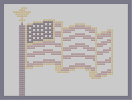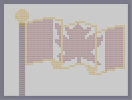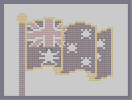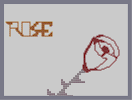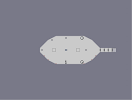N Statue Stars and Stripes oooOOooh Canada... Down Under! Every Beauty has it's Thorns Claustrophobia

Pages: (0)

### Ok

It doesnt look to good but you got to love the halo 2

### yeys

gooooooooooood keep makin halo art

### hmm

its ok, oddly cluttered and maybe some poorly placed objects...

but its still pretty good...

4.5, nice job

### Wow

If only I could do that

5/5

### duh its laggy

you don't have to load it

But i love it.
4/5